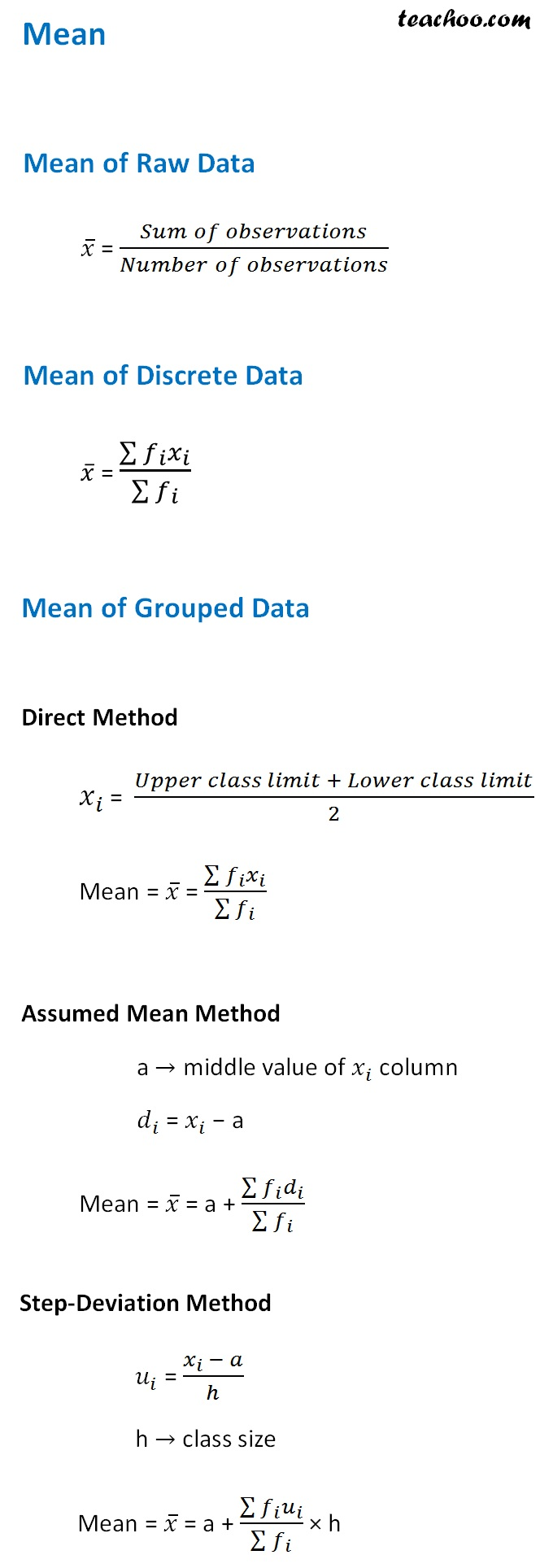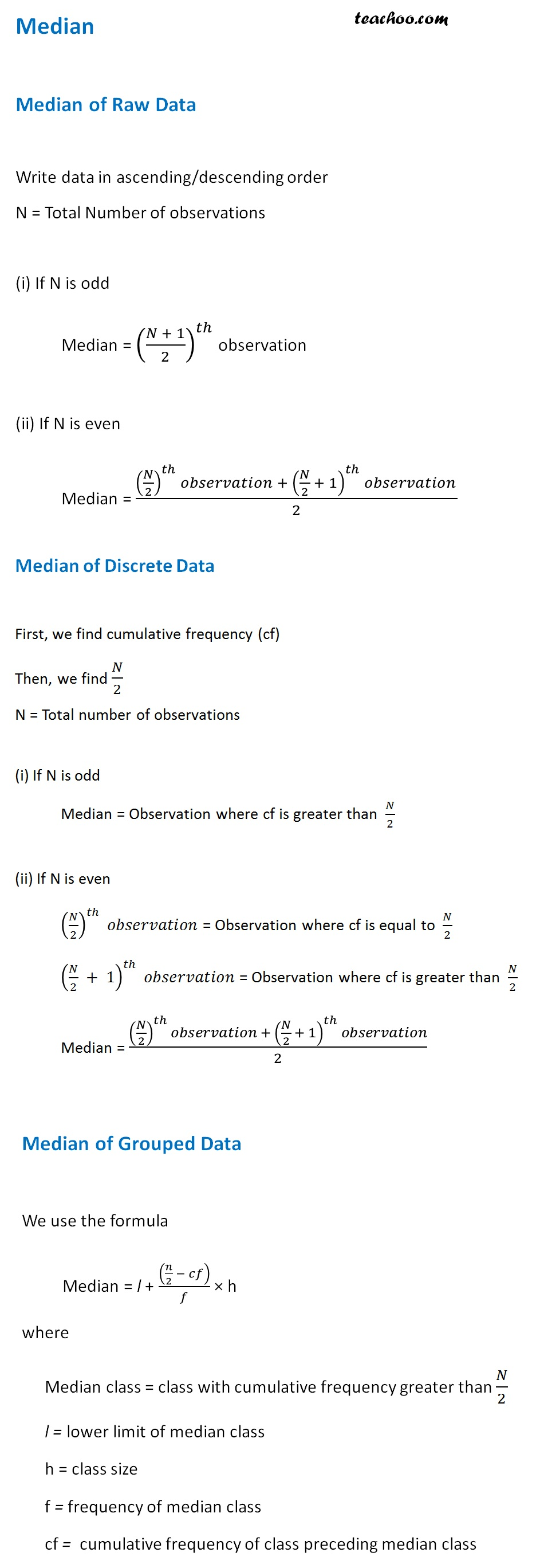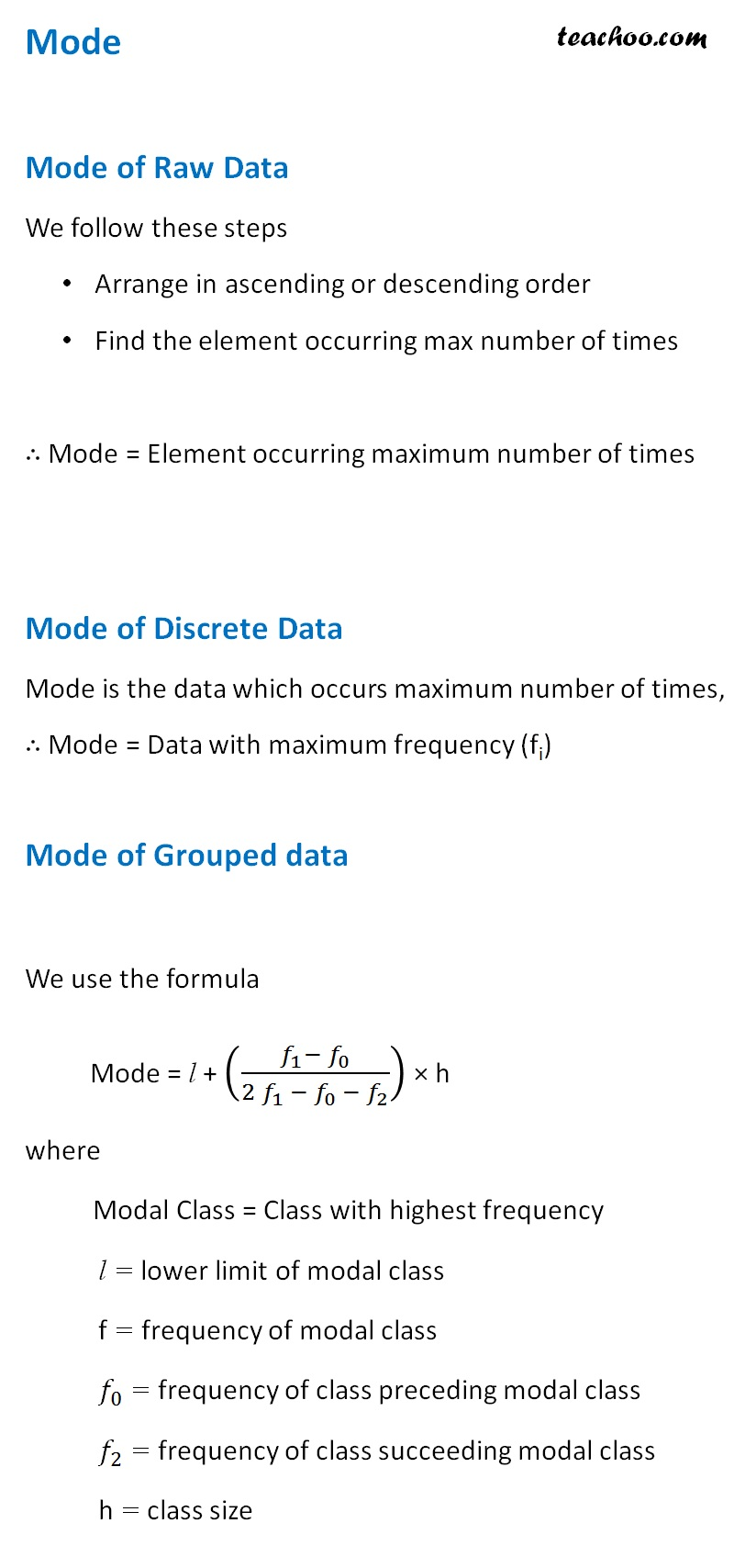Less than , more than ogive

Chapter 13 Class 10 Statistics
Concept wise

Here, we list all formulas that are required for Class 9 and 10 Exams

The formula list includes

• Range
• Mean
• Mean of Raw Data
• Mean of Discrete Data
• Mean of Grouped Data
• Median
• Median of Raw Data
• Median of Discrete Data
• Median of Grouped Data
• Mode
• Mode of Raw Data
• Mode of Discrete Data
• Mode of Grouped Data

## Range

Range = Maximum value – Minimum Value

For data

5, 10, 15, 18, 21, 24, 24, 13, 2, 9, 9, 18, 18, 32, 28, 3, 14, 25, 3, 27

Maximum value = 32

Minimum value = 2

Thus,

Range = 32 – 2 = 30

## Mean of Raw, Discrete and Grouped Data## Median of Raw, Discrete and Grouped DataWe can also find median using Ogive curve

We draw less than and more than ogive,

and their intersection is the median

## Mode of Raw, Discrete and Grouped DataLearn in your speed, with individual attention - Teachoo Maths 1-on-1 Class

### Transcript

Mean of Raw Data 𝑥 ̅ = (𝑆𝑢𝑚 𝑜𝑓 𝑜𝑏𝑠𝑒𝑟𝑣𝑎𝑡𝑖𝑜𝑛𝑠)/(𝑁𝑢𝑚𝑏𝑒𝑟 𝑜𝑓 𝑜𝑏𝑠𝑒𝑟𝑣𝑎𝑡𝑖𝑜𝑛𝑠) Mean of Discrete Data 𝑥 ̅ = (∑▒〖𝑓_𝑖 𝑥_𝑖 〗)/(∑▒𝑓_𝑖 ) Mean of Grouped Data Direct Method 𝑥_𝑖 = (𝑈𝑝𝑝𝑒𝑟 𝑐𝑙𝑎𝑠𝑠 𝑙𝑖𝑚𝑖𝑡 + 𝐿𝑜𝑤𝑒𝑟 𝑐𝑙𝑎𝑠𝑠 𝑙𝑖𝑚𝑖𝑡)/2 Mean = 𝑥 ̅ = (∑▒〖𝑓_𝑖 𝑥_𝑖 〗)/(∑▒𝑓_𝑖 ) Assumed Mean Method a → middle value of 𝑥_𝑖 column 𝑑_𝑖 = 𝑥_𝑖 − a Mean = 𝑥 ̅ = a + (∑▒〖𝑓_𝑖 𝑑_𝑖 〗)/(∑▒𝑓_𝑖 ) Step-Deviation Method 𝑢_𝑖 = (𝑥_𝑖 − 𝑎)/ℎ h → class size Mean = 𝑥 ̅ = a + (∑▒〖𝑓_𝑖 𝑢_𝑖 〗)/(∑▒𝑓_𝑖 ) × h Median Median of Raw Data Write data in ascending/descending order N = Total Number of observations (i) If N is odd Median = ((𝑁 + 1)/2)^𝑡ℎ observation (ii) If N is even Median = ((𝑁/2)^𝑡ℎ 𝑜𝑏𝑠𝑒𝑟𝑣𝑎𝑡𝑖𝑜𝑛 + (𝑁/2 + 1)^𝑡ℎ 𝑜𝑏𝑠𝑒𝑟𝑣𝑎𝑡𝑖𝑜𝑛)/2 Median of Discrete Data First, we find cumulative frequency (cf) Then, we find 𝑁/2 N = Total number of observations (i) If N is odd Median = Observation where cf is greater than 𝑁/2 (ii) If N is even (𝑁/2)^𝑡ℎ 𝑜𝑏𝑠𝑒𝑟𝑣𝑎𝑡𝑖𝑜𝑛 = Observation where cf is equal to 𝑁/2 (𝑁/2 + 1)^𝑡ℎ 𝑜𝑏𝑠𝑒𝑟𝑣𝑎𝑡𝑖𝑜𝑛 = Observation where cf is greater than 𝑁/2 Median = ((𝑁/2)^𝑡ℎ 𝑜𝑏𝑠𝑒𝑟𝑣𝑎𝑡𝑖𝑜𝑛 + (𝑁/2 + 1)^𝑡ℎ 𝑜𝑏𝑠𝑒𝑟𝑣𝑎𝑡𝑖𝑜𝑛)/2 Median of Grouped Data We use the formula Median = l + ((𝑛/2 − 𝑐𝑓))/𝑓 × h where Median class = class with cumulative frequency greater than 𝑁/2 l = lower limit of median class h = class size f = frequency of median class cf = cumulative frequency of class preceding median class Mode Mode of Raw Data We follow these steps Arrange in ascending or descending order Find the element occurring max number of times ∴ Mode = Element occurring maximum number of times Mode of Discrete Data Mode is the data which occurs maximum number of times, ∴ Mode = Data with maximum frequency (fi) Mode of Grouped data We use the formula Mode = l + ((𝑓_1− 𝑓_0)/(2 𝑓_1 − 𝑓_(0 )− 𝑓_2 )) × h where Modal Class = Class with highest frequency l = lower limit of modal class f = frequency of modal class 𝑓_0 = frequency of class preceding modal class 𝑓_2 = frequency of class succeeding modal class h = class size